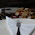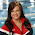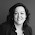## Saturday, June 26, 2010

### Lesson 16: Technician Class Exam Prep

Here is lesson 16.  This lesson may prove to be the toughest one yet in preparing for the Technician Class exam.  This lesson covers the T5B questions dealing with metric conversions and calculating decibels (dB).  Don't worry I've broken down what you need to know.  There is a metric conversion chart in the links section, but for the purposes of the exam I will lay down what you need to memorize.

Keep in mind that the prefix in a metric unit (kilo, mega, pico, etc) describes the size of a particular measurement to the standard unit of measure.  So if the standard unit of measure is watts, a kilowatt is 1000 watts.  These metric prefixes help us deal with really big and really small numbers.  Here are the ones you need to memorize:

Pico = 1/1,000,000,000,000 (1 trillionth) of the standard unit (Hz, Amperes, Volts, etc.)
Micro = 1/1,000,000 of the standard unit (Hz, Amperes, Volts, etc.)
Milli = 1/1000 of the standard unit (Hz, Amperes, Volts, etc.)
Kilo = 1000 of the standard unit (Hz, Amperes, Volts, etc.)
Mega = 1,000,000 of the standard unit (Hz, Amperes, Volts, etc.)

To find how many kHz go into a MHz, one way to find the answer is to break the number down into Hz.  A kHz is 1000 Hz.  a MHz is 1,000,000 Hz.  So to find out how many kHz go into a MHz, all you do is divide the bigger unit into the smaller unit.  1,000,000/1000 = 1000 so 1 MHz is equal to 1000 kHz.

To find how many MHz 3525 kHz is, you can break the numbers down into the base unit (Hz) as well.  So 3525 kHz is equal to 3,525,000 Hz and a MHz is equal to 1,000,000 Hz.  Divide 3,525,000 by 1,000,000 and you get 3.525 MHz.  It's a lot easier if you've ever been taught to multiply and divide by powers of 10.  If you remember, the conversion chart in the links section has the powers of 10 listed.

The other piece of memorization you need to do is the formula for calculating decibels (dB).  Bels are often used to calculate the amount of change in noise or power.  Decibels are the units used most often.  A decibel is 1/10th of a Bel.  The way this is used is it calculates the amount of change from one power or noise level to another.  Logarithms are used in the formula so it would be wise to find yourself a good scientific calculator.  On the exam, the questions deal with changes in power.

The formula you need to memorize is:

dB = 10log(P1/P0
Where P0 is the reference power (where you're starting from) and P1 is the power being compared to the reference power (where you are ending).  So if you were to calculate the change in dB from an increase in power from 3 watts to 10 watts, P0 would be the 3 Watts (where you're starting) and P1 would be 10 Watts (where you're ending).  Substitute these numbers into the formula and that's it.  It's also important to note that if the change in power is a decrease (10 watts to 3 watts, for example) the answer on the calculator will be a negative number.  Since we are dealing with the amount of change, the answer will always be positive.  It is like calculating distance.  If you drove 20 miles and them backtracked 5 miles, the total distance traveled is 25 miles.  Just because you backtracked doesn't mean those 5 miles are subtracted from the 20 miles.  Change is the same way.  A decrease in power only means the change is happening down vice up.  Clear as mud?

If you have any questions, comments, or suggestions (there might be a few in this one...I am a rotten math teacher) please leave them in the comments box.  Until the next lesson...

73,
Andy
KE4GKP

#### 6 comments:

1.Andy, my name is Pete and I am 13 years of age. This is very diffucult for me but I can use a calculator easily. I found a salution to figure out the answer to this question, What is the approximate amount of change, measured in decibels, of a power decrease from 12 watts to 3 watts?- 6 dB. I remember that 3 times 2 is 6 and 12 divided by 2 is 6. Easy!

1.You have almost stumbled upon something even more useful. There are two really, really useful things to remember when working with db.

1. if you double/halve the power that is a 3db change

2. if you increase/decrease the power by 10x that is a 10db change.

So, when going from 3 watts to 12 watts you are doubling the power twice, 3watts to 6 watts and then 6 watts to 12 watts. So you are increasing it 3db + 3db = 6db.

2.So far, the number of points and ideas given here are actually what I was looking for from the last few hours, hopefully this would proved to be the best guide, if further information taken out from the same platform to assist in more efficient way. Visit http://best-college-essay.com/ for best essays.

3.This comment has been removed by a blog administrator.

4.Interesting topic for a blog. I have been searching the Internet for fun and came upon your website. Fabulous post. Thanks a ton for sharing your knowledge! It is great to see that some people still put in an effort into managing their websites. I'll be sure to check back again real soon. Walter

5.If you are interested in the idea of using free, environmental friendly energy to power your household, and you want to have a solar panel array installed, one of the most basic requirements you need to keep track of is the number of solar panels that you need. There are many factors that would determine just how many you would need to use, and how many you will actually be able to buy. Some of these include the results of your energy consumption target calculations (how much energy your household appliances and devices need in... Nano Hydromx Energy Saving